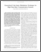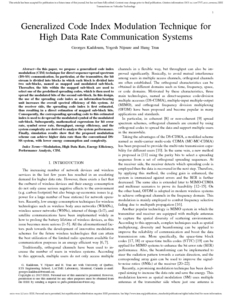A showcase of ÉTS researchers’ publications and other contributions
 SEARCH

Generalized code index modulation technique for high data rate communication systems

Kaddoum, Georges, Nijsure, Yogesh et Tran, Hung. 2016. « Generalized code index modulation technique for high data rate communication systems ». IEEE Transactions on Vehicular Technology, vol. 65, nº 9. pp. 7000-7009.
Compte des citations dans Scopus : 41.Preview
PDF
Generalized-Code-Index-Modulation-Technique-for-High-Data-Rate-Communication-Systems.pdf

Abstract

In this paper, we propose a generalized code index modulation (CIM) technique for direct sequence-spread spectrum (DS-SS) communication. In particular, at the transmitter, the bit stream is divided into blocks in which each block is divided into two sub-blocks, named as mapped and modulated sub-block. Thereafter, the bits within the mapped sub-block are used to select one of the predefined spreading codes, which is then used to spread the modulated bits of the second sub-block. In this design, the use of the spreading code index as an information-bearing unit increases the overall spectral efficiency of this system. At the receiver side, the spreading code index is first estimated, thus resulting in a direct estimation of mapped sub-block bits. Consequently, the corresponding spreading code to this estimated index is used to de-spread the modulated symbol of the modulated sub-block. Subsequently, mathematical expressions for bit error rate, symbol error rate, throughput, energy efficiency, and the system complexity are derived to analyze the system performance. Finally, simulation results show that the proposed modulation scheme can achieve higher data rate than the conventional DSSS system, with lower energy consumption and complexity.

Item Type: Peer reviewed article published in a journal
Professor:
ProfessorView Item